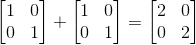Hi, I have a question about invertible matrix. If A is invertible does adding the identity matrix to it still make it invertible.

In other words, A +I = B , it B still invertible? Why?

Thanks.

Dick
Homework Helper
-I is invertible. Adding I to it gives you something pretty noninvertible.

-I is invertible. Adding I to it gives you something pretty noninvertible.The question actually is:
Solve for X. If you need to invert a matrix explain why that matrix is invertible.

A,B,X, are nbyn matrices. A,X, A-AX are invertible.

(A-AX)^-1 = X^-1 B

So I got X = BA(I + BA) ^-1
I'm just not sure why (I + BA) is invertible.
Thanks for the help

Dick
Homework Helper
The question actually is:
Solve for X. If you need to invert a matrix explain why that matrix is invertible.

A,B,X, are nbyn matrices. A,X, A-AX are invertible.

(A-AX)^-1 = X^-1 B

So I got X = BA(I + BA) ^-1
I'm just not sure why (I + BA) is invertible.
Thanks for the help

You got there by showing BA=(1+BA)X, right? If X-AX is invertible and X is invertible then B is invertible from your original equation, yes? Now if B is invertible, A is invertible and X is invertible, then (1+BA) is invertible. If you have an equation like J*K=L and two of the three matrices are invertible, then the third is invertible. And haven't you got the BA and (1+BA)^(-1) factors in reverse order?

Last edited:
Makes sense, Thanks!
Edit: yes, the ordering should be the other way.

Hi, is there anyway you could go over how you got to the form X= BA(I+BA)^-1 from the original equation?

Dick